# Representation Expectation

This post is by Round Rock ISD Elementary Math Coordinator, Brian Bushart. Connect with Brian on Twitter and join him in #mtbos and #elemmathchat

When you think of the STAAR test, is this the type of wordy word problem that comes to mind: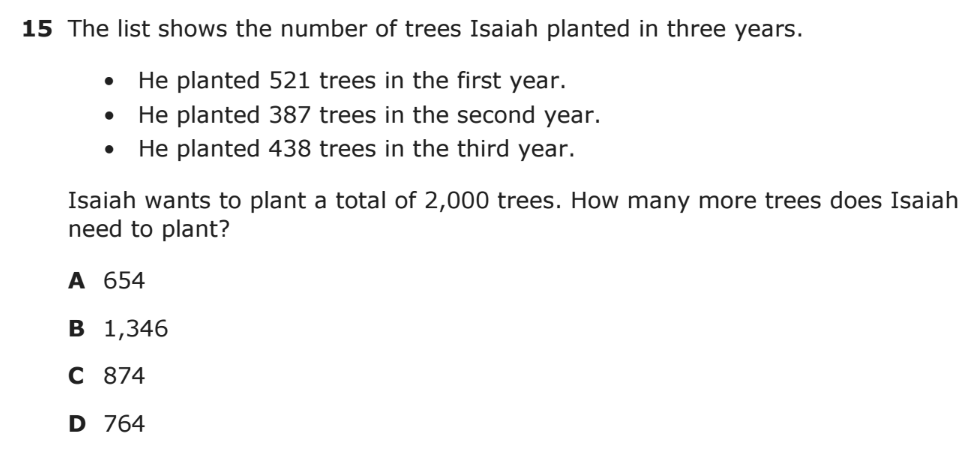Source: Texas Education Agency (STAAR 2016, Grade 4, Question 15)

What if I told you that questions like this — questions that require students to make sense of a “real-life” situation, select the appropriate operation(s), and perform the calculation(s) correctly to find the answer — what if I told you these kinds of questions only make up about 30% of the grade 3 STAAR and 40% of the grades 4 and 5 STAAR tests? That’s not even half the test in any of these grades!

So what’s being assessed by the remaining 60–70% of the STAAR test?

After reviewing all of the questions from the released 2016 STAAR tests for grades 3, 4, and 5, it became clear that very many of them require students to analyze and make connections among representations — symbols, pictures, diagrams, tables, graphs, and language.

What might surprise you is that answering these questions often requires little to no computation. Can you imagine that? A math test where many questions don’t require computation?!

Let’s look closely at one of these questions.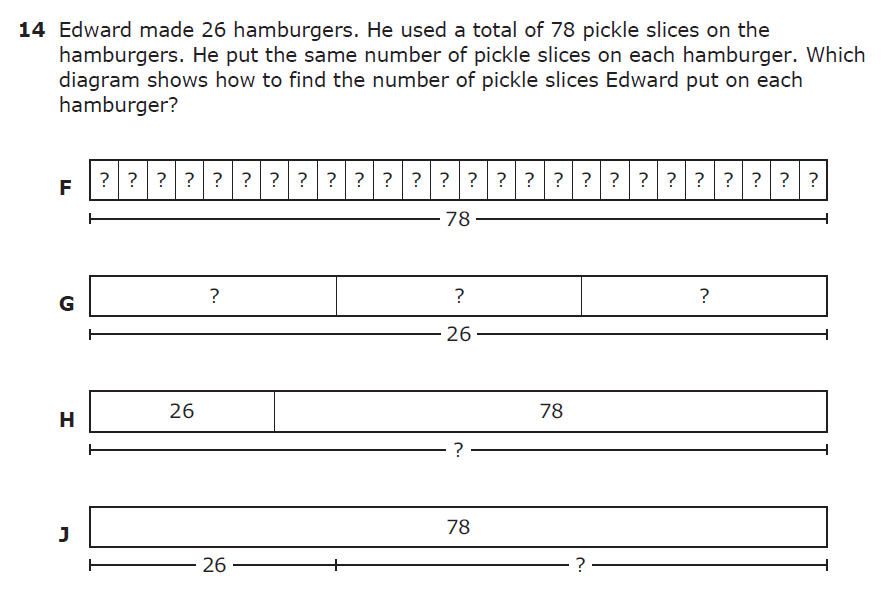Source: Texas Education Agency (STAAR 2016, Grade 3, Question 14)

This question requires students to connect two different types of representations.

• In the question, students are provided a language representation.
• In each answer choice, students are provided a strip diagram representation.

In order to answer this question correctly — assuming students aren’t guessing — students must analyze and connect one of the strip diagram representations with the language representation.

How does one go about doing this?

Students need to analyze both types of representations and look for features that map between them. The first thing students will likely notice is that both the language and strip diagram representations contain the same two numbers — 26 and 78. Two of the strip diagrams contain both numbers and two of the strip diagrams contain only one of the numbers. If a student is just connecting the representations at a surface level, he might choose choice H or J because 26 and 78 both appear in those strip diagrams.

However, if the student understands strip diagrams, the student might go deeper and notice that choices F and G are equal-part strip diagrams and choices H and J are part-whole strip diagrams. Knowing that, the student might go back into the language representation to see which type of strip diagram maps to the situation.

If a student does this, she should quickly recognize that choices H and J are not possible correct answers because this situation is not about adding 26 hamburgers and pickles to find a total number of…something, like choice H represents. It is also not a case of removing 26 hamburgers from 78 pickles as shown in choice J because that doesn’t even make any sense.

The student is now left with choices F and G. Choice F is probably the craziest looking strip diagram here because there are just so many boxes with question marks. The total of 78 is being divided into so many equal groups. However, if you analyze the language representation, that makes sense because the 78 pickles are being put on so many hamburgers, 26 to be precise. If you count the number of boxes in the strip diagram, there just happen to be 26 boxes, one for each hamburger. The only thing we don’t know, as represented by the question mark, is the number of pickles on each burger. As we’re told in the language representation, we know it’s the same amount on each hamburger.

Whew! Thankfully this kind of reasoning happens a lot faster in our minds than it does writing it all down, but I wanted to be very explicit in the kinds of analytical thinking and connection making required to answer this type of question correctly.

And remember, questions like this — questions that require students to analyze and make connections between representations — made up a bulk of the questions on the 2016 STAAR test.

Here are a few more examples you should take a few minutes to analyze on your own. Here are some questions to help guide you:

1. What type(s) of representation is in the question?
2. What type(s) of representation is in the answer choices?
3. What features do students need to attend to when analyzing and connecting the correct representation in the answer choices to the representation in the question?
4. What kind of instruction needs to occur in the classroom to prepare students to be successful with these kinds of questions?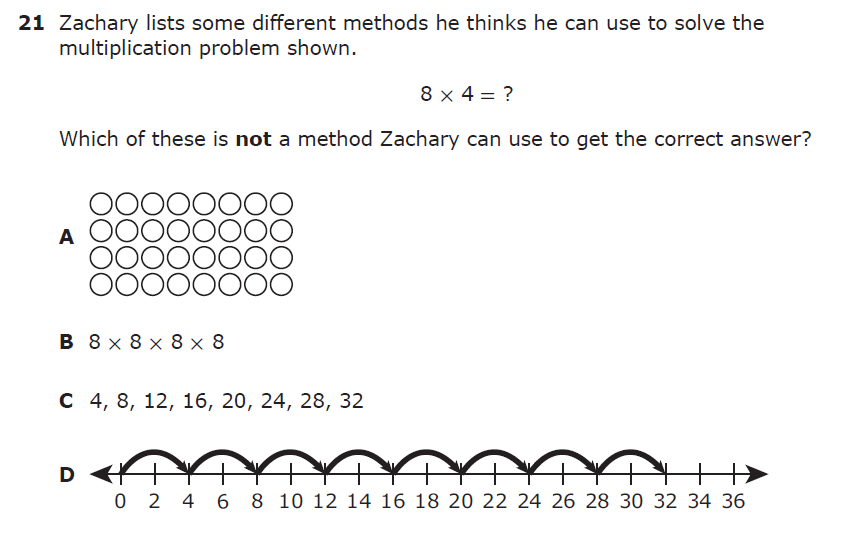Source: Texas Education Agency (STAAR 2016, Grade 3, Question 21)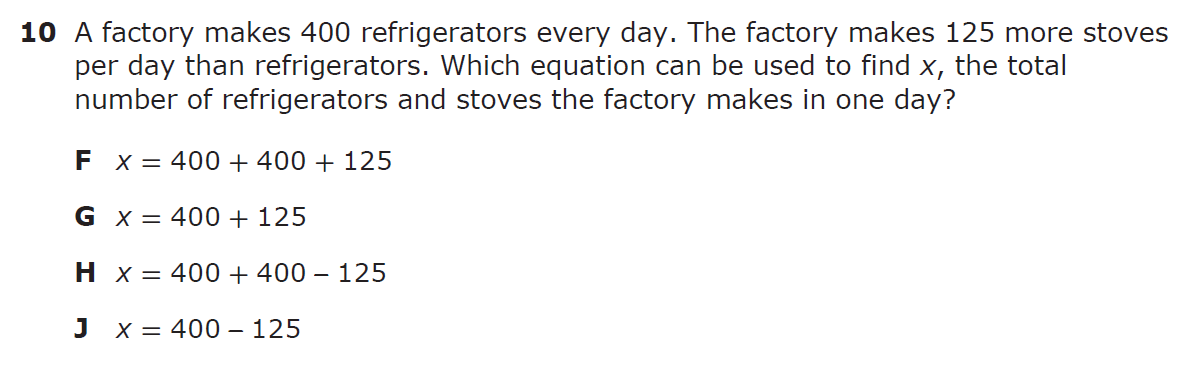Source: Texas Education Agency (STAAR 2016, Grade 4, Question 10)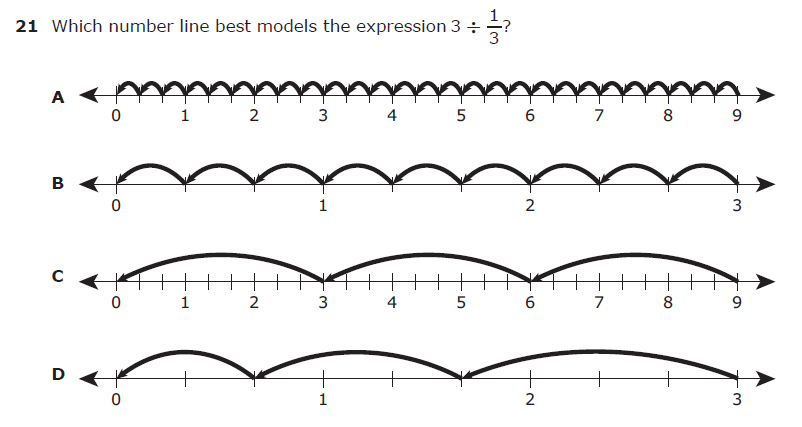Source: Texas Education Agency (STAAR 2016, Grade 5, Question 21)

So why are so many questions like this? Isn’t it enough to know how to calculate the product of 8 and 4 or the quotient of 3 and 1/3?

Not anymore.

When the math TEKS were revised in 2012, the verb “represent” was included all over the place. In addition, specific representations were called for in the TEKS. For example, TEKS 3.4E says: represent multiplication facts by using a variety of approaches such as repeated addition, equal-sized groups, arrays,area models, equal jumps on a number line, and skip counting.

If you go back up and look at question 21 from the grade 3 STAAR, you’ll see the answer choices include four of those approaches — an array, skip counting, equal jumps on a number line, and what would have been repeated addition if the representation were correct.

Our standards (TEKS) and assessment (STAAR) hold students accountable for more than procedural fluency with computation. Students are required to have the conceptual understanding to back it up, and one of the ways that conceptual understanding is built is through robust experiences where students create, use, analyze, communicate with, and connect multiple representations, as evidenced in not only in the content standards but also in the process standards at each grade level K-12:

• TEKS 1D communicate mathematical ideas, reasoning, and their implications using multiple representations, including symbols, diagrams, graphs, and language as appropriate;
• TEKS 1E create and use representations to organize, record, and communicate mathematical ideas;
• TEKS 1F analyze mathematical relationships to connect and communicate mathematical ideas;

Going forward, we need to be thoughtful about the kinds of learning experiences we’re designing for our students to ensure that they are simultaneously building conceptual understanding and procedural fluency. Careful and intentional unit planning are essential to ensure students are building robust mathematical understandings that will serve them well on STAAR and support them as they continue to grow as learners and doers of mathematics.

## You are now leaving Round Rock ISD

Round Rock ISD provides links to web sites of other organizations in order to provide visitors with certain information. A link does not constitute an endorsement of content, viewpoint, policies, products or services of that web site. Once you link to another web site not maintained by Round Rock ISD, you are subject to the terms and conditions of that web site, including but not limited to its privacy policy.

You will be redirected to

Click the link above to continue or CANCEL